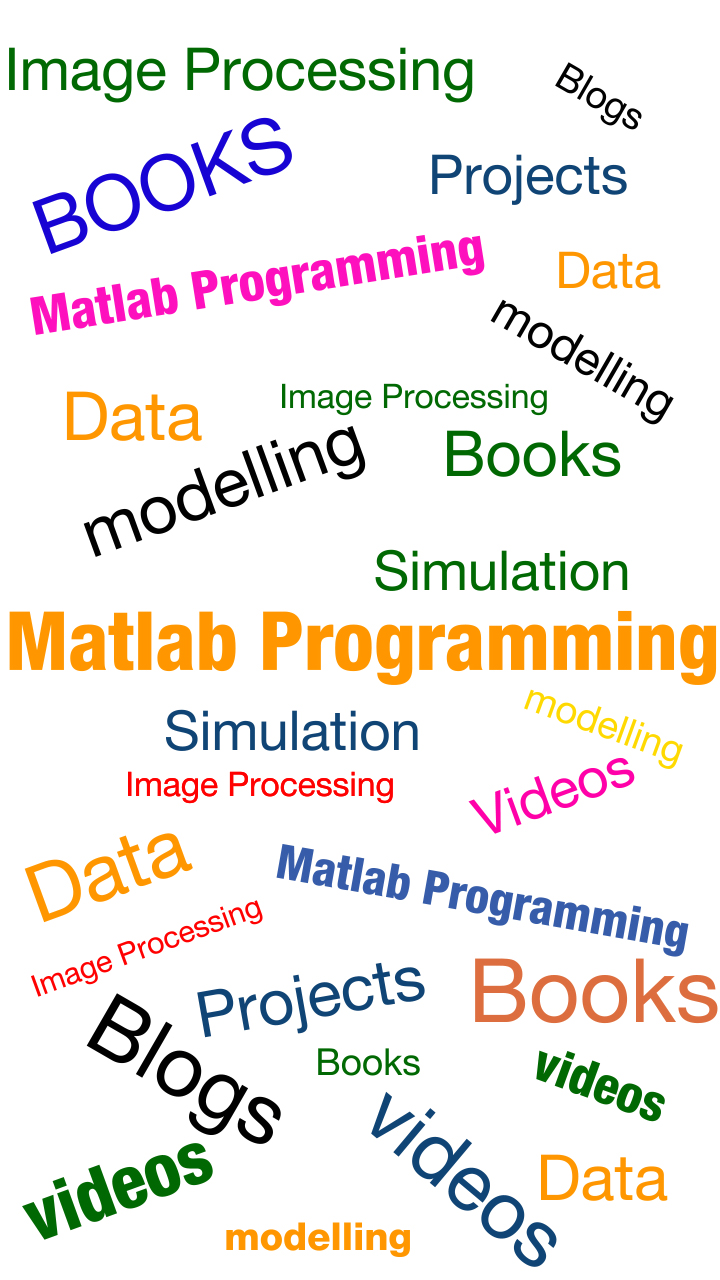### Blogs / VideosIntroduction to MATLAB Programming

Here’s a list of video tutorials published by MIT Open Courseware. These tutorials are best to use by a first timer of MATLAB.

MATLAB for Beginners

Here’s a complete for beginners to learn MATLAB. This tutorial will introduce with basic functions, commands and concepts used for working in MATLAB.

This is an online course on MATLAB provided by Mathworks. This course is not free and involves a certain amount of fee. This course introduces the extensive usage of MATLAB using image processing, machine learning, parallel computing and more similar concepts.

Complete Course on YouTube – Introduction to MATLAB

Here’s a complete list of tutorials on MATLAB available for free. These videos allow you to get familiar with various MATLAB commands and data manipulation techniques.

Modelling and Simulations using MATLAB

This is an online course on MATLAB modelling and simulations. It covers wide range of MATLAB components which includes introduction to MATLAB components, statistics & image processing, basic & advanced problem solving toolbox and various application using MATLAB

Coursera – Introduction to Programming with MATLAB

This is a MOOC on MATLAB programming provided by coursera. This course is best suited for beginners who have no prior experience in computer science. This course teaches various functions and commands used in MATLAB.

This youtube channel is dedicated to teaching MATLAB through various informative tutorial videos. More than just learning, you’ll also find interviews, conversation with best MATLAB experts spread across the world.